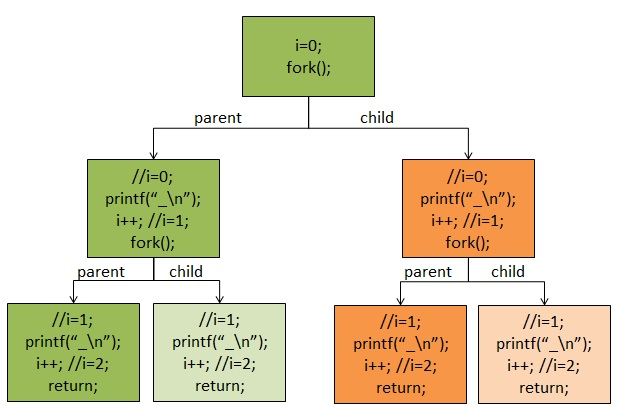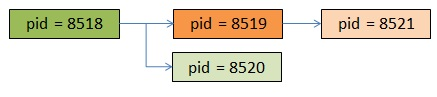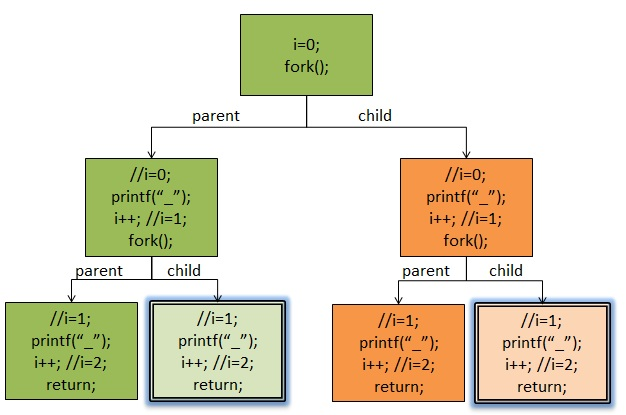```#include <stdio.h>
#include <sys/types.h>
#include <unistd.h>

int main(void)
{
int i;
for(i=0; i<2; i++){
fork();
printf("-");
}

wait(NULL);
wait(NULL);

return 0;
}```

printf(“-\n”);

printf(“-“);
fflush(stdout);

```#include <stdio.h>
#include <sys/types.h>
#include <unistd.h>
int main(void)
{
int i;
for(i=0; i<2; i++){
fork();
//注意：下面的printf有“\n”
printf("ppid=%d, pid=%d, i=%d \n", getppid(), getpid(), i);
}
sleep(10); //让进程停留十秒，这样我们可以用pstree查看一下进程树 return 0;
}```

```ppid=8858, pid=8518, i=0
ppid=8858, pid=8518, i=1
ppid=8518, pid=8519, i=0
ppid=8518, pid=8519, i=1
ppid=8518, pid=8520, i=1
ppid=8519, pid=8521, i=1

\$ pstree -p | grep fork
|-bash(8858)-+-fork(8518)-+-fork(8519)---fork(8521)
|            |            `-fork(8520)```